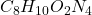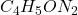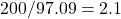## An empirical formula calculation gives a molar ratio of 1.0 oxygen, 4.8 hydrogen, 4.1 carbon and 1.8 nitrogen. If the molecular mass is appr

Question

An empirical formula calculation gives a molar ratio of 1.0 oxygen, 4.8 hydrogen, 4.1 carbon and 1.8 nitrogen. If the molecular mass is approximately 200 amu, what is the molecular formula

in progress 0
5 months 2021-08-10T06:00:34+00:00 1 Answers 3 views 0

## Answers ( )Explanation:

Hello there!

In this case, according to the given information about the empirical formula of this compound, we can infer that these mole ratios can be rounded to the following whole numbers in order to find the empirical formula:Whose molar mass is 97.09 amu and thus, the ratio of the molecular to the empirical molar mass is:Which is almost a factor of 2; and therefore, the resulting molecular formula is:Regards!